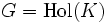Left transiter of normal is characteristic

Statement

Statement with symbols

Let$H \le K$ be a subgroup. The following are equivalent:

1.$H$ is a Characteristic subgroup (?) of$K$
2. If$G$ is a group containing$K$ such that$K$ is a Normal subgroup (?) of$G$, then$H$ is also a normal subgroup of$G$.

Property-theoretic statement

The left transiter of the subgroup property of normality is the subgroup property of characteristicity. In other words:

• Characteristic$*$ Normal$\le$ Normal

Every characteristic subgroup of a normal subgroup is normal (the$*$ here is for the composition operator).

• If$p$ is such that:$p *$ Normal$\le$ Normal

Then$p \le$ Characteristic

Definitions used

Characteristic subgroup

• Hands-on definition: A subgroup$H$ of a group$G$ is termed characteristic in$G$ if for any automorphism$\sigma$ of$G$,$\sigma(H) = H$.
• Definition using function restriction expression: A subgroup$H$ of a group$G$ is termed characteristic in$G$ if it has the following function restriction expression:

Automorphism$\to$ Automorphism

In other words, every automorphism of$G$ restricts to an automorphism of$H$.

Normal subgroup

• Hands-on definition: A subgroup$H$ of a group$G$ is termed normal in$G$ if for every$g \in G$,$gHg^{-1} = H$.
• Definition using function restriction expression: A subgroup$H$ of a group$G$ is termed normal in$G$ if it has the following function restriction expression:

Inner automorphism$\to$ Automorphism

In other words, every inner automorphism of$G$ restricts to an automorphism of$H$.

Related facts

Related left residual computations

All these use the fact that inner automorphism to automorphism is right tight for normality.

Some examples with a somewhat different flavor:

Upper hooks and related facts

• Normal upper-hook fully normalized implies characteristic: If$H \le K \le G$ are such that$H$ is normal in$G$ and$K$ is fully normalized in$G$, then$H$ is characteristic in$K$.
• Characteristically simple and normal fully normalized implies minimal normal

Facts used

1. Characteristic of normal implies normal (used for the more direct part of the proof).
2. Inner automorphism to automorphism is right tight for normality

Proof

Hands-on proof

Given: A subgroup$H$ of a group$K$.

To prove: The following are equivalent:

1.$H$ is characteristic in$K$.
2. For every group$G$ in which$K$ is normal,$H$ is normal in$G$.

Proof: (1) implies (2) follows from fact (1). We now prove (2) implies (1).

For the group$K$, let$\operatorname{Hol}(K)$ denote the holomorph of$K$.$\operatorname{Hol}(K)$ is the semidirect product of$K$ with$\operatorname{Aut}(K)$. Observe that every automorphism of$K$ lifts to an inner automorphism of$\operatorname{Hol}(K)$, and further, that$K$ is a normal subgroup of$\operatorname{Hol}(K)$.

Now let$H$ be a subgroup of$K$ with the property that whenever$K$ is normal in a group$G$, so is$H$. We will show that$H$ is characteristic in$K$.

Take$G = \operatorname{Hol}(K)$. Clearly$K$ is normal in$G$. Now, let$\sigma \in \operatorname{Aut}(K)$. Clearly, there is an inner automorphism of$G$ whose restriction to$K$ is$\sigma$ (namely, conjugation by the element of$\operatorname{Aut}(K)$ that is$\sigma$). But$H$ is normal in$G$, so every inner automorphism of$G$ must leave$H$ invariant, and hence$\sigma$ must leave$H$ invariant. This shows that$H$ is invariant under all automorphisms of$K$, and hence, is a characteristic subgroup.

Property-theoretic proof

By fact (2), the function restriction expression:

Inner automorphism$\to$ Automorphism

is a right tight function restriction expression for normality. In other words, for every automorphism of a group, there is a bigger group in which it is normal, such that the automorphism extends to an inner automorphism in that bigger group. Combining this with fact (3), we see that the left transiter of normality is the property:

Automorphism$\to$ Automorphism

which is indeed the property of being a characteristic subgroup.